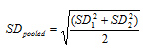# Cohen's Effect Size Calculator for T-test#Effect Size Calculator

An online Cohen's d effect size calculator.

Effect Size Equations Formula

Formula Used to Calculate Cohens d is

d = (M1 - M2 ) / SDpooledWhere,

M1 = mean of group 1,

M2 = mean of group 2,

SD1 = standard deviation of group 1,

SD2 = standard deviation of group 2,

SDpooled = pooled standard deviation.

## Cohen's scale

Our calculator uses the following table of synonyms for the descriptors

Effect SizeDescriptor
less than 0.2Trivial
greater than 0.2Small
greater than 0.5Moderate
greater than 0.8Large##Age Calculators ►

Horse Age Calculator , Cow Age Calculator , Cat Age Calculator , Dog Age Calculator, Human Age Calculator 100 Days Old Calculator Date Difference Calculator